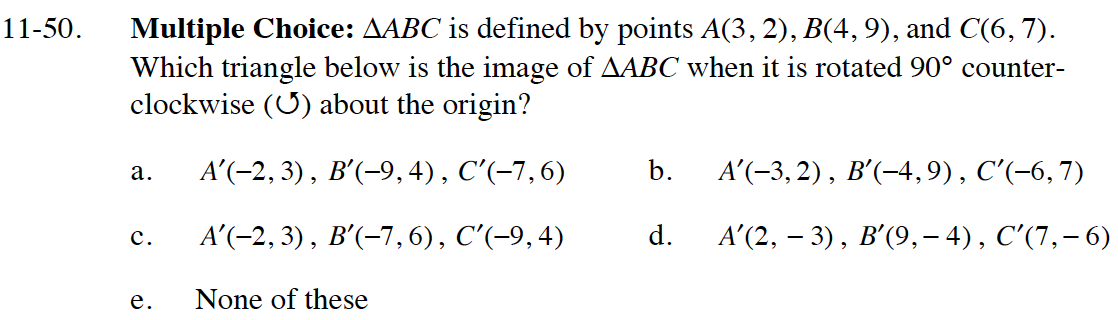Home > CC4 > Chapter 11 > Lesson 11.2.1 > Problem11-50

11-50.

Multiple Choice: ΔABC is defined by points A(3, 2), B(4, 9), and C(6, 7). Which triangle below is the image of ΔABC when it is rotated 90° counter-clockwise (↺) about the origin? 11-50 HW eTool (Desmos). Homework Help ✎

 a. A′(−2, 3), B′(−9, 4), C′(−7, 6) b. A′(−3, 2), B′(−4, 9), C′(−6, 7) c. A′(−2, 3), B′(−7, 6), C′(−9, 4) d. A′(2, −3), B′(9, −4), C′(7, −6) e. none of theseThe original coordinates of point A were (3, 2). How are the coordinates of point A' related to point A?

Use the table in the eTool below to enter the points from each choice above. When adding points to the table below, copy the first point again at the end to connect.
Click the link at the right for the full version: Int1 11-50 HW eTool.